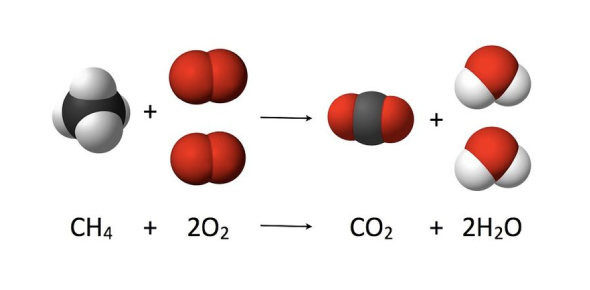# Chemistry Quiz: Stoichiometry MCQs

10 Questions | Attempts: 199SettingsStoichiometry in chemistry is the quantitative relationship between reactants and various products in a chemical reaction. And here is a quiz with ten multiple choice questions to test your knowledge of the topic. So, without further ado, let's get started.

• 1.
Which of the following compound is more soluble in non polar solvent?
• A.

Graphite

• B.

Glucose

• C.

Sulphur

• D.

Lithium fluoride

• 2.
Which of the following compound in solution form is best for titrant and its own indicator in redox titration ?
• A.

I2

• B.

NaOCl

• C.

K2Cr2O7

• D.

KMnO4

• 3.
The equilibrium constant for reaction of Fe2+ and SCN-1 as given below can be determined by
• A.

Chromatography

• B.

Spectroscopy

• C.

Conductance

• D.

Ion exchange

• 4.
On reaction with dil HCl, which of the following compound produce a gas at 25oC that is more denser than air ?
• A.

PbNO3

• B.

Zn

• C.

NaHCO3

• D.

NaBr

• 5.
A 20.00 mL of a Ba(OH)2 solution is treated with 0.245 M HCl. If 27.15 mL of HCl is completely used for this reaction, what is the molarity of the Ba(OH)2 solution??
• A.

0.166 M

• B.

0.180

• C.

0.333

• D.

0.666d

• 6.
Ionic hydride reacts with water  to give
• A.

Basic solution and oxygen gas

• B.

Acidic solution and hydrogen gas

• C.

Acidic solution and oxygen gas

• D.

Basic solution and hydrogen gas

• E.

None of the above

• 7.
The number of moles of Na+ ions present in 20 ml of 0.40 M Na3PO4, are:
• A.

0.20

• B.

0.024

• C.

0.0080

• D.

0.050

• 8.
The percentage of oxygen (by mass) in Al2SO4.18H2O
• A.

28.8

• B.

26.6

• C.

48.2

• D.

72.0

• 9.
When the equation give below is balanced with whole number coefficients, the coefficient for H+(aq) is __Mn2+(aq)  + __ BiO3 (aq) + __H +(aq) → __Bi3+(aq) + __MnO4 (aq) + __H2O(l)
• A.

4

• B.

6

• C.

8

• D.

14

• 10.
The number of O2 molecules in the 2.5 g of O2 inhaled by the average person in one minute are?
• A.

1.8 × 1022

• B.

3.6 × 1022

• C.

4.7 × 1022

• D.

5.4 × 1022

## Related TopicsBack to top
×

Wait!
Here's an interesting quiz for you.